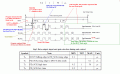# Hx711 interfacing with PIC16F877A

#### Hajji

Joined Mar 4, 2022
3
Hi..

I am interfacing 24 bit ADC ( HX711 ) with micro controller, after reading datasheet specially 'Reference Driver（C )' , i didn't understand : Count=Count^0x800000;
Can anyone please explain this instruction. And how micro controller transform the data from hx711 to decimal number
Thank You.

#### ericgibbs

Joined Jan 29, 2010
15,525
Hi Hajii
"^" means "Binary XOR Operator"

E

The first Bit read is the SIGN Bit, it is weighted as the MSBit.

When the Weight Count is Positive ie: above Zero then this Bit is '0'
If a Negative Weight Count, this Bit is '1'.
Action of: Count = Count ^ 0x800000, Sets the MSBit, the SIGN Bit to '0'

So the unsigned long Count is the Value, to which you need to adjust, to the Actual weight, by using the Calibration factor of the Scale weights

Ref the image:Last edited:

#### spitsnsparkin

Joined Jun 19, 2011
18
Hi Hajji

In assembly language, toggling the MSB (^ 0x800000 in your case) is a quick way to convert between signed numbers and offset-binary (like the exponents used in floating-point numbers). For MCU's that lack signed math opcodes, by converting the signed numbers to offset-binary, you can quickly perform a comparison (or subtraction) and simply evaluate the carry and zero flags for magnitude information. For a quick example with 16-bits integers:
Decimal: -32768, 0, +32767
Signed: 0x8000, 0x0000, 0x7FFF
After toggling the MSB:
Offset-Binary: 0x0000, 0x8000, 0xFFFF